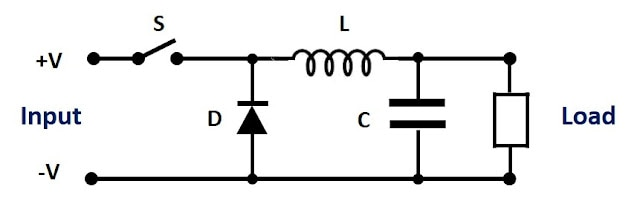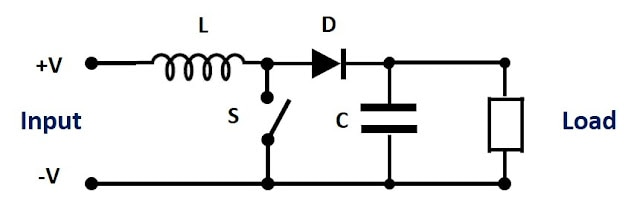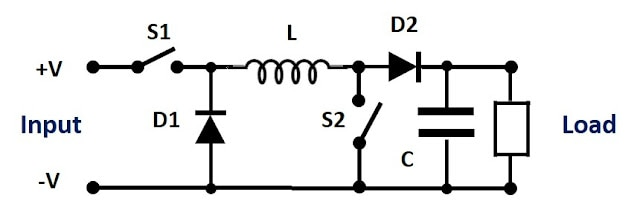TDK-Lambda Americas Blog

# What are buck, boost and buck-boost DC-DC converters?

Buck Boost DC-DC Converters Technology

July 31, 2020

Buck, boost and buck-boost DC-DC converters are widely used in power supply designs, and have been for many years. They are popular because of their simplicity, low cost and high efficiency. There is no transformer used in the design, and as such, there is no isolation between the input voltage and the output voltage.

In this post I will explain the differences between these three converters and a high level review on how they function. (As a note the simplified schematics do show diodes and switches, in reality FETs are used as synchronous rectifiers to reduce losses and improve efficiency.)

A buck converter reduces voltage and the output voltage is lower than the input voltage. See Figure 1.Figure 1: Buck converter

When transistor S is turned on, energy is stored in inductor L as the current flows to the load and capacitor C is charged. When S is off, the energy stored in L is released and current flows into the load and circulates via diode D. Capacitor C also provides energy to the load. This is repeated at a high frequencies, greater than 100,000 times a second. The length of the time S is turned on defines the output voltage.

A boost converter increases voltage and the output voltage is higher than the input voltage. See Figure 2.Figure 2: Boost converter

When transistor S is turned on, current flows through inductor L, through transistor S back to the input. During this period energy is stored in the inductor. When transistor S is off, the inductor acts a voltage source in series with the input voltage. The inductor’s stored energy is circulated through diode D to the load. This charges capacitor C to a higher level than the input voltage. Again, the length of the time S is turned on defines the output voltage.

This boost converter topology is also used in most Power Factor Control (PFC) sections of AC-DC power supplies. The control IC is different of course, as its purpose is to ensure the AC input current drawn is sinusoidal in shape. At high line voltages greater than 240Vac the DC input may be higher than the voltage on capacitor C. This will reduce the PFC boost converter’s performance and the power factor will be degraded slightly.

A buck-boost converter is a combination of a buck and boost converter. The output voltage can be higher or lower than the input voltage. See Figure 3.Figure 3: Buck-boost converter

As you can see the circuit is more complicated and has more components. S2, L, and D2 is the boost converter (S1 being on) and S1, L and D1 the buck section (S2 being off).

Many manufacturers like TDK-Lambda offer both buck and buck-boost converters. With less components and complexity, a buck converter will offer a lower cost, higher efficiency and either a smaller package or more output power.Figure 4: TDK-Lambda’s i7C 300W buck-boost DC-DC converterPAGE TOP